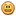NOTIFICATION: These examples are provided for educational purposes. The use of this code and/or information is under your own responsibility and risk. The information and/or code is given ‘as is’. I do not take responsibilities of how they are used. You are welcome to point out any mistakes in my posting and/or leave a comment.

Previously, we saw how to check the type of the function map:

```Hugs> :t map
map :: (a -> b) -> [a] -> [b]
```

Haskell have a peculiar way to express types.

One way to interpreting this is the following:

```map :: (a -> b) -> [a] -> [b]
map ::   {- is the function name -}
(a -> b) {- This means that map takes a function as a argument -}
-> [a]   {- This means that map also takes an array of element type a as an argument -}
-> [b]   {- And return an array of elements type b -}
However, Haskell work using currying, therefore the another way to see this is the following:
map :: (a -> b) -> [a] -> [b]
map ::   {- is a function -}
(a -> b) {- that takes a function as a argument and return a new function (we called map_1) -}
-> [a]   {- This new function (map_1) will take an array of type a as an argument -}
-> [b]   {- and will return an array of element of type b -}
In Haskell, currying is supported in order to support first-class functions. For example:

Lets assume a function takes two arguments; however, only one argument will be supplied
The function will be “curried” which means that  it will produce a function of one argument (one that is not supplied)
Therefore we could see it in this way:
fn :: a -> b -> c
where fn(a) yields a new function
fn' :: b -> c
in which fn'(b) is called to produce c

For example, lets say we wish to obtain the square root of the values 2 and 3 which are in a list, and return a new list with the results:
[2, 3] => [1.41..., 1.73...]
We can use map together with the function sqrt (square root)!
If we check the type of sqrt we will find that:
Hugs> :t sqrt
sqrt :: Floating a => a -> a
This is a function that takes an element of type a as argument and return an element of type a as a result.
If we use it together with map, we obtain:
Hugs> :t map sqrt
map sqrt :: Floating a => [a] -> [a]
This means that when map takes sqrt function as a parameter, it will create a new function that will take an array of type a and return an array of type a.
Now let see it in action:
Hugs> map sqrt [2, 3]
[1.4142135623731,1.73205080756888]
Now, lets analyse what could be happening internally in Haskell when this command line is executed:

The code of map is the follow:
-- Map and append
map :: (a -> b) -> [a] -> [b]
map f []     = []
map f (xs) = f x : map f xs

The code of sqrt is the follow:
class  (Fractional a) => Floating a  where
...
exp, log, sqrt      :: a -> a
(**), logBase       :: a -> a -> a
...
x ** y           =  exp (log x * y)
sqrt x           =  x ** 0.5

When we execute:
> map sqrt [2, 3]

map sqrt [2, 3]
match with
> map f (xs)

Therefore,
f x : map f xs
is executed in which f is sqrt, x is the first element of the list, and xs is the rest of the list
sqrt 2 : map sqrt 

Then
map sqrt 
match again
f x : map f xs
and is executed again to  obtain
sqrt 2 : sqrt 3 : map sqrt []

map sqrt []
match
map f []     = []
obtaining
[]
Then we obtain
sqrt 2: sqrt 3 : []

Finally, the functions are executed and we obtain
1.4142135623731 : 1.73205080756888 : []
which returns
[1.4142135623731, 1.73205080756888]

The following are some examples (fn means function) of types:

fn is just a value:
fn :: x

fn return a list of ys
fn :: x -> [y]

fn return a typle of (y, z)
fn :: x -> (y, z)

fn is a higher order function. It takes a function for argument (b->c) as an input an return a function Foo
fn :: (b -> c) -> Foo

fn is an input/output action tha return an int value
fn :: x -> IO Int